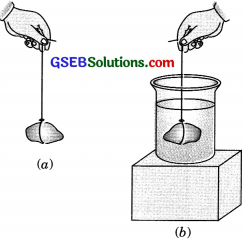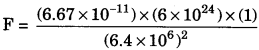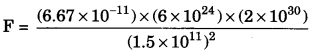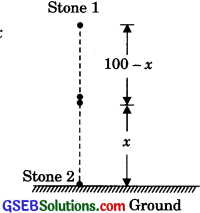# GSEB Solutions Class 9 Science Chapter 10 Gravitation

Gujarat Board GSEB Textbook Solutions Class 9 Science Chapter 10 Gravitation Textbook Questions and Answers, Notes Pdf.

## Gujarat Board Textbook Solutions Class 9 Science Chapter 10 Gravitation

### Gujarat Board Class 9 Science Gravitation InText Questions and Answers

Page 134

Question 1.
State the universal law of gravitation.
Every particle in the universe attracts every other particle with a force, which is directly proportional to the product of their masses and inversely proportional to the square of the distance between the two masses. The direction of force is along the line joining the two particles.”

Question 2.
Write the formula to find the magnitude of the gravitational force between the Earth and an object on the surface of the Earth.
Take, M = mass of Earth
m = mass of object
r = distance between object and centre of object
Gravitational force between object and the Earth is given by,
F = $$\frac{\mathrm{G} \mathrm{Mm}}{r^{2}}$$Page 136

Question 1.
What do you mean by tree fall?
Whenever objects fall towards the Earth under gravitational force alone, we say that the objects are in free fall.

Question 2.
What do you mean by the acceleration due to gravity?
The acceleration with which a body falls towards the Earth due to Earth’s gravitational pull is known as acceleration due to gravity.

Page 138

Question 1.
What are the differences between the mass of an object and its weight?
Mass:

• The mass of a body is the quantity of matter contained in it.
• The mass of an object is constant and does not change from place to place.
• SI unit of mass is the kilogram (kg).

Weight:

• The weight of an object is the force with which it is attracted to the Earth.
• The weight of an object changes from place to place because the acceleration due to gravity varies with position and location.
• SI unit of weight is Newton (N).

Question 2.
Why is the weight of an object on the moon $$\frac {1}{6}$$th its weight on the Earth?
The weight of an object is given by, w = mg. Acceleration due to gravity on the moon is $$\frac {1}{6}$$ th of that of the Earth.
Hence weight is also $$\frac {1}{6}$$th.Page 141

Question 1.
Why it is difficult to hold a school bag having a strap made of a thin and strong string?
For a thin strap, the area is less and pressure is more since the pressure is inversely proportional to area. Hence it is difficult to hold a bag with a thin strap.

Question 2.
What do you mean by buoyancy?
When a body is partially or wholly immersed in a fluid, an upward force acts on it which is called upthrust or buoyant force.

Question 3.
Why does an object float or sink when placed on the surface of the water?
When an object is placed in water two forces act on it – the weight of the object and buoyant force. If the weight of the object is balanced by buoyant force, then the object floats. If the weight of the object is greater than the buoyant force, then the object sinks.

Page 142

Question 1.
You find your mass to be 42 kg on a weighing machine. is your mass more or less than 42 kg?
If the measured mass is 42 kg, then the true mass is slightly greater than 42 kg. The measured value is less than true value due to the effect of buoyant force which acts vertically upwards.

Question 2.
You have a bag of cotton and an iron bar, each indicating a mass of 100 kg when measured on a weighing machine. In reality, one is heavier than the other. Can you say which one is heavier and why?
The cotton bag is heavier than the iron bar. The volume of cotton is much more than the volume of iron, so the buoyant force of air on cotton is much more than that of air on an iron bar. This is because, weight measured = Actual weight – Buoyant force or Actual weight = Weight measured + Buoyant force.In-Text Activities Solved

(Textbook Page 131)

Activity 10.1

Discussion and conclusion

• Stone moves in a circular path.
• The direction of motion after release is tangent to the circular path at that point.(Textbook Page 134)

Activity 10.2
Discussion and conclusion
1. Gravitational force acts downward. It provides retardation to the stone due to which velocity of the stone decreases continuously during upward motion and becomes zero at the topmost point. After that stone starts moving in the downward direction.

(Textbook Page 135)

Activity 10.3
Discussion and conclusion

• Air resistance on paper is more than that of stone.
• In a vacuum, both will reach the floor of the glass jar at the same time as air resistance is zero in, vacuum.(Textbook Page 140)

Activity 10.4
Discussion and conclusion

• Gravitational force is acting downward and buoyant force is acting upwards.
• As we are pushing the bottle downward, the immersed volume increases and more water is displaced. Hence buoyant force also increases.
• When the bottle is completely immersed in water, the buoyant force is greater than the gravitational force which results in bouncing back of the bottle to the surface.

(Textbook Page 140)

Activity 10.5
Discussion and conclusion
Iron nail sinks. The weight of the iron nail is greater than the upthrust on the iron nail or the density of iron nail is greater than the density of water.

(Textbook Page 141)

Activity 10.6
Discussion and conclusion
The iron nail will sink into the water while the cork floats over its surface. This is because the density of iron is more than that of water whereas that of the cork is less than that of water.(Textbook Page 141)

Activity 10.7
Discussion and conclusion
As the stone is slowly dipped into the water, the elongation of the string or the reading on the balance will decrease. This decrease
in elongation or balance reading will continue as more and more of the stone is dipped into water, the elongation or reading will become least and will remain constant if the stone is lowered still deeper. The decrease in elongation is due to an increase in the upthrust. As the immersed volume increases upthrust increases.### Gujarat Board Class 9 Science Gravitation Textbook Questions and Answers

Question 1.
How does the force of gravitation between two objects change when the distance between them is reduced to half?
According to Newton’s law of gravitation, force between masses is given by F =$$\frac{\mathrm{G} m_{1} m_{2}}{r^{2}}$$
F = $$\frac{1}{r^{2}}$$
If the distance becomes hali force becomes four times.
As F = $$\frac{\mathrm{G} m_{1} m_{2}}{(r / 2)^{2}}=\frac{4 \mathrm{G} m_{1} m_{2}}{r^{2}}$$ = 4F
∴ F = 4F

Question 2.
Gravitational force acts on all objects in proportion to their masses. Why then, a heavy object does not fall faster than a light object?
All objects fall on the ground with constant acceleration, called acceleration due to gravity. It is constant and does not depend on the mass of an object.Question 3.
What is the magnitude of the gravitational force between the Earth and a 1 kg object on its surface?
[Mass of the Earth is 6 x 10 kg and radius of the Earth is 6.4 x 106 m]
Given,
Massofbody, m = 1kg
MassofEarth, M = 6 x 1024kg
Radius of Earth, r = 6.4 x 106 m
Force, F = ?
The gravitational force between two bodies is given by
F = $$\frac{GMm}{r^{2}}$$F= 9.8N

Question 4.
The Earth and the moon are attracted to each other by gravitational force. Does the Earth attract the moon with a force that is greater or smaller or the same as the force with which the moon attracts the Earth? Why?
According to the universal law of gravitation, two objects attract each other with equal force, but in opposite directions. The Earth attracts the moon with equal force with which the moon attracts the Earth.

Question 5.
If the moon attracts the Earth, why does the Earth not move towards the moon?
The force exerted by the Earth on the moon is used in changing the path of the moon from a straight line to circular. The moon attracts the Earth with the same force. But the Earth is too heavy to move towards the moon.

Question 6.
What happens to the force between two objects, if –

1. the mass of one object is doubled?
2. the distance between the objects is doubled and tripled?
3. the masses of both objects are doubled?

From universal law of gravitation, force between two masses is given by
F = $$\frac{\mathrm{G} m_{1} m_{2}}{r^{2}}$$

1. When mass of one object is doubled, force becomes twice
let m’1 = 2m1
New force F’ = $$\frac{G\left(2 m_{1}\right)\left(m_{2}\right)}{r^{2}}=\frac{2 G m_{1} m_{2}}{r^{2}}$$ = 2F
∴ F = 2F2. When distance between the objects is doubled, force becomes one fourth of its previous value.
Initially f F = $$\frac{\mathrm{G} m_{1} m_{2}}{r^{2}}$$
Finally distance (separation),
r’ = 2r
F = $$\frac{\mathrm{Gm}_{1} m_{2}}{(2 r)^{2}}=\frac{\mathrm{G} m_{1} m_{2}}{4 r^{2}}=\frac{\mathrm{F}}{4}$$
∴ F’ = $$\frac {F}{4}$$

When distance between the objects is tripled, the force becomes one ninth of its previous value.
Initially force, F = $$\frac{\mathrm{G} m_{1} m_{2}}{r^{2}}$$
Finally distance (separation),
r’ = 3r
F = $$\frac{\mathrm{Gm}_{1} m_{2}}{(3 r)^{2}}=\frac{\mathrm{G} m_{1} m_{2}}{9 r^{2}}$$
∴ F’ = $$\frac {F}{9}$$

3. When masses of both objects are doubled, force becomes four times
Initially, F = $$\frac{\mathrm{G} m_{1} m_{2}}{r^{2}}$$
Now, m’1= 2m1 and m’2 = 2m2
= F’ = $$\frac{\mathrm{G} m_{1}^{\prime} m_{2}^{\prime}}{r^{2}}=\frac{G\left(2 m_{1}\right)\left(2 m_{2}\right)}{r^{2}}=\frac{4 \mathrm{G} m_{1} m_{2}}{r^{2}}$$ = 4F
∴ F’ = 4F

Question 7.
What is the importance of the universal law of gravitation?
The universal law of gravitation is important as it accounts for –

1. The motion of the planets around the Sun.
2. The motion of the moon and other artificial satellites around the Earth.
3. The tides formed by the gravitational pull of the moon.
4. Force of gravity of the Earth and other planets.

Question 8.
What is the acceleration of free fall?
Acceleration of free fall is the acceleration produced when a body falls under the influence of the force of gravitation of the Earth alone. It is denoted by and its value on the surface of Earth is 9.8 ms-2.

Question 9.
What do we call the gravitational force between the Earth and an object?
The gravitational force between the Earth and an object is called the Earth’s gravity. The force on an object due to Earth’s gravity is the weight.

Question 10.
Amit buys a few grains of gold at the poles as per the instruction of one of his friends. He hands over the same when he meets him at the equator. Will the friend agree with the weight of gold bought? If not, why?
[Hint: The value of g is greater at the poles than at the equator]
Amit’s friend will not agree with the weight of the gold bought.
As the weight of an object on Earth is given by –
w = mg
Acceleration due to gravity at the pole is greater than at the equator. Hence Amit’s friend will not agree.Question 11.
Why does a sheet of paper fall slower than one that is crumpled into a ball?
A sheet of paper will fall slower than the one that is crumpled into a ball. This is because air offers resistance due to friction to the motion of the falling object. The resistance offered by air to the sheet of paper is more than the resistance offered by air to the paper ball because the sheet has a larger area.

Question 12.
The gravitational force on the surface of the moon is only as strong as the gravitational force on the Earth. What is weight in newtons of a 10 kg object on the moon and on the Earth?
Mass of object, m = 10 kg
Weight of object on Earth, we = mge = 10 x 9.8 = 98 N
Weight of object on moon, wm = mgm = 10 x $$\frac {9.8}{6}$$ = 16.3 N

Question 13.
A ball Is thrown vertically upwards with a velocity of 49 m/s. Calculate

1. the maximum height to which it rises,
2. the tota] time it takes to return to the surface of the Earth.

Given, Initial velocity u = 49 m/s
Taking upward direction positive acceleration, a = – g = – 9.8 m/s2

1. For maximum height
applying equation, υ2 – u2 = 2as
(0)2 – (49)2 = 2(- 9.8)h
h = 122.5 m

2. For time of flight
Total time = time of ascent + time of descent
= t + t = 2t
Fortimeofascenttake υ = 0
Applying υ = u + at
0 = 49 + (-9.8)t
∴ t = 5 s
Totaltime = 2t = 10 s

Question 14.
A stone is released from the top of a tower of height 19.6 m. Calculate its final velocity just before touching the ground.
Given, Height = h = 19.6 m
a = 9.8 m/s2
Applying υ2 – u2 = 2as
⇒ υ2 – 02 = 2 x 9.8 x 19.6
∴ u = 19.6 m/sQuestion .15.
A stone is thrown vertically upward with an initial velocity of 40 m/s. Taking g – 10 m/s2, find the maximum height reached by the stone. What is the net displacement and the total distance covered by the stone?
Given,
Initial velocity, u = 40 m/s
Final velocity, υ = m/s
Acceleration, a = -g = – 10 m/s2
Taking upward direction positive for maximum height,
Applying equation,
u2 – u2 = 2as
02– (40)2 = 2(-10) x h
∴ h = 80 m
Therefore, total distance covered by the stone during ita upward and downward journey = 80 m + 80 m = 160 m. As the final position coincides with its initial position, net displacement = 0.

Question 16.
Calculate the force of gravitation between the Earth and the Sun, given that the mass of the Earth -6 x 10301 kg and of the Sun = 2 x 1011 kg. The average distance between the two is 1.5 x 1011
Given,
Mass of the Sun, M = 2 x l030 kg
Mass of the Earth  M = 6 x 1024 kg
The average distance between the Earth and the Sun,
r = 1.5 x 1011 m
Applying Newton’s law of gravitation, the force between the Earth and the Sun is given by
F = $$\frac{\mathrm{GM}_{e} \mathrm{M}_{6}}{r^{2}}$$
orF = 3.57 x 1022 NQuestion 17.
A stone is allowed to (all from the top of a tower 100 m high and at the same time another stone is projected vertically upwards from the ground with a velocity of 25 m/s. Calculate when and where the two stones will meet.
Given, h = 100 m
Let the two stones meet after t seconds at a point P which is at a height x
above the ground as shown in Figure.
Taking upward direction as negative,For stone 1
u = 0, h = (100 – x)m
a = g = 9.8 m/s2
From s = ut + $$\frac {1}{2}$$ at2
or, (100 – x) = 0 x t + $$\frac {1}{2}$$ (9.8)t2
∴ 100 – x = 4.9t2 ………..(i)

For stone 2
u = 25 m/s, h = x
a = -g = -9.8 m/s2
From s = ut +$$\frac {1}{2}$$ at2
or, x = 25t + $$\frac {1}{2}$$(-9.8)t2
∴ x = 25t – 4.9 t2 ……..(ii)
From equation (i) and (ii),
or,  x = 25 t – 100 + x
∴ t = 4s
Putting the value oft in equation (i)
100 – x = 4.9 x (4)2
∴ x = 21.6m
∴ 100 – x = 100 – 21.6 = 78.4m
Therefore, the stones will meet after 4s at a height of 78.4 m from the top of the tower or 21.6 m from the base of the tower.

Question 18.
A ball thrown up vertically returns to the thrower after 6 s. Find
(a) the velocity with which it was thrown up.
(b) the maximum height it reaches, and
(c) its position after 4s.
Given, total time = 6 s
acceleration, a = -g = – 9.8 m/s2
(taking upward direction as negative)(a) Applying equation s = ut + $$\frac {1}{2}$$ at2
As object returned to its initial position its displacement is zero, s = 0
0 = u x 6 + $$\frac {1}{2}$$(- 9.8) (6)2
6u = 176.4
∴ u = 29.4 m/s

(b) Total time = time of ascent + time of descent
6s = t + t
or, 2t = 6s
∴  t = 3s
Applying s = ut +$$\frac {1}{2}$$ at2
h = 29.4 x 3 + $$\frac {1}{2}$$ (-9.8)(3)2
∴ h = 44.lm

(c) In the first 3s, the ball will rise vertically upward, then in the next 3s it will fall towards the Earth.
Therefore position after 4s = Distance covered in is in its downward journey.
Applying  s = ut + $$\frac {1}{2}$$ at2
u = 0,
taking t = 1s
s = ut + $$\frac {1}{2}$$ at2
⇒ s = (0) + $$\frac {1}{2}$$ (-9.8) (1)2
∴ s = 4.9 m

Question 19.
In what direction does the buoyant force on an object immersed in a liquid act?
The buoyant force on an object immersed in a liquid always acts vertically upwards.

Question 20.
Why does a block of plastic released under water come up to the surface of the water?
The upthrust or buoyant force acting on the plastic block is more than the weight of the object. Due to it upward, the force plastic block comes up to the surface of the water.Question 21.
The volume of 50 g of a substance is 20 cm3. If the density of water is 1 g cm, will the substance float or sink?
Given,
volume of substance, u = 20 cm3
mass of substance, m = 50 g
density of water, dw = 1 g/cm3
density of substance, ds = $$\frac {m}{υ}$$ = $$\frac{50 \mathrm{g}}{20 \mathrm{cm}^{3}}$$ = 2.5 g/cm3
The substance will sink, as density of the substance is greater than the density of water (ds> dm).

Question 22.
The volume of a 500 g sealed packet is 350 cm3. Will the packet float or sink in water if the density of water is 1 g cm3? What will be the mass of the water displaced by this packet?
density of packet, dp = $$\frac {m}{υ}$$ = $$\frac{500 \mathrm{g}}{350 \mathrm{cm}^{3}}$$ = 1.428 g/cm3